How to Remove a Column by name and index using Dplyr Package in R

• Last Updated : 21 Jul, 2021

In this article, we are going to remove columns by name and index in the R programming language using dplyr package.

Dataset in use: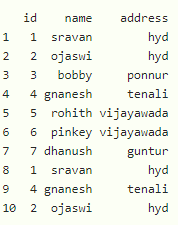Remove a column by using column name

We can remove a column with select() method by its column name.

Syntax:

select(dataframe,-column_name)

Where, dataframe is the input dataframe and column_name is the name of the column to be removed.

Example: R program to remove a column

R

 # load the library library(dplyr)    # create dataframe with 3 columns  # id,name and address data1=data.frame(id=c(1,2,3,4,5,6,7,1,4,2),                                      name=c('sravan','ojaswi','bobby',                         'gnanesh','rohith','pinkey',                         'dhanush','sravan','gnanesh',                         'ojaswi'),                                      address=c('hyd','hyd','ponnur','tenali',                            'vijayawada','vijayawada','guntur',                            'hyd','tenali','hyd'))    # remove name column print(select(data1,-name))    # remove id column print(select(data1,-id))

Output: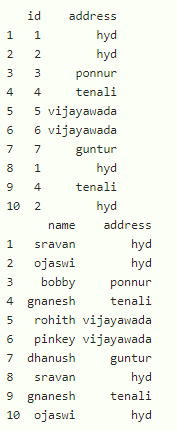Remove multiple columns by using column name

We can remove a column with select() method by its column name

Syntax:

select(dataframe,-c(column_name1,column_name2,.,column_name n)

Where, dataframe is the input dataframe and -c(column_names) is the collection of names of the column to be removed.

Example: R program to remove multiple columns

R

Output: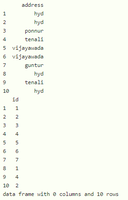Remove a column by using column index

We can remove a column with select() method by its column index/position. Index starts with 1.

Syntax:

select(dataframe,-column_index)

Where, dataframe is the input dataframe and column_index  is the position of the column to be removed.

Example: R program to remove particular column

R

 # load the library library(dplyr)    # create dataframe with 3 columns # id,name and address data1=data.frame(id=c(1,2,3,4,5,6,7,1,4,2),                                      name=c('sravan','ojaswi','bobby',                         'gnanesh','rohith','pinkey',                         'dhanush','sravan','gnanesh',                         'ojaswi'),                                      address=c('hyd','hyd','ponnur','tenali',                            'vijayawada','vijayawada','guntur',                            'hyd','tenali','hyd'))    # remove name column by its position print(select(data1,-2))    # remove address column by its position print(select(data1,-3))

Output: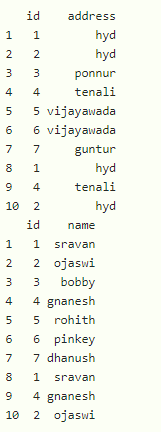Remove multiple columns by using column index

We can remove a column with select() method by its column index/position. Index starts with 1.

Syntax:

select(dataframe,-c(column_index1,column_index2,.,column_index n)

Where, dataframe is the input dataframe and c(column_indexes) is the position of the columns to be removed.

Example: R program to remove multiple columns by position

R

 # load the library library(dplyr)    # create dataframe with 3 columns # id,name and address data1=data.frame(id=c(1,2,3,4,5,6,7,1,4,2),                                      name=c('sravan','ojaswi','bobby',                         'gnanesh','rohith','pinkey',                         'dhanush','sravan','gnanesh',                         'ojaswi'),                                      address=c('hyd','hyd','ponnur','tenali',                            'vijayawada','vijayawada','guntur',                            'hyd','tenali','hyd'))    # remove name and id  columns by # its position print(select(data1,-c(1,2)))

Output: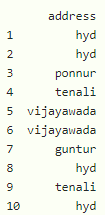My Personal Notes arrow_drop_up
Recommended Articles
Page :